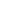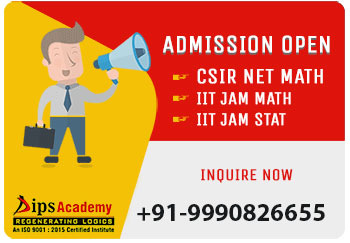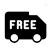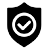## CUET PG 2023 Maths

#### Offers##### News & Events# CUET PG Maths Syllabus 2023

#### Syllabus for Mathematics (PGQP27)

Algebra: Groups, subgroups, Abelian groups, non-abelian groups, cyclic groups, permutation groups; Normal subgroups, Lagrange's Theorem for finite groups, group homomorphism and quotient groups, Rings, Subrings, Ideal, Prime ideal; Maximal ideals; Fields, quotient field.
Vector spaces, Linear dependence and Independence of vectors, basis, dimension, linear transformations, matrix representation with respect to an ordered basis, Range space and null space, rank-nullity theorem; Rank and inverse of a matrix, determinant, solutions of systems of linear equations, consistency conditions. Eigenvalues and eigenvectors. Cayley-Hamilton theorem. Symmetric, Skew symmetric, Hermitian, Skew-Hermitian, Orthogonal and Unitary matrices.

Real Analysis: Sequences and series of real numbers. Convergent and divergent sequences, bounded and monotone sequences, Convergence criteria for sequences of real numbers, Cauchy sequences, absolute and conditional convergence; Tests of convergence for series of positive terms-comparison test, ratio test, root test, Leibnitz test for convergence of alternating series.
Functions of one variable: limit, continuity, differentiation, Rolle's Theorem, Cauchy’s Taylor's theorem. Interior points, limit points, open sets, closed sets, bounded sets, connected sets, compact sets; completeness of R, Power series (of real variable) including Taylor's and Maclaurin's, domain of convergence, term-wise differentiation and integration of power series.
Functions of two real variable: limit, continuity, partial derivatives, differentiability, maxima and minima. Method of Lagrange multipliers, Homogeneous functions including Euler's theorem.

Complex Analysis: Functions of a complex Variable, Differentiability and analyticity, Cauchy Riemann Equations, Power series as an analytic function, properties of line integrals, Goursat Theorem, Cauchy theorem, consequence of simply connectivity, index of a closed curves. Cauchy’s integral formula, Morera’s theorem, Liouville’s theorem, Fundamental theorem of Algebra, Harmonic functions.

Integral Calculus: Integration as the inverse process of differentiation, definite integrals and their properties, Fundamental theorem of integral calculus. Double and triple integrals, change of order of integration. Calculating surface areas and volumes using double integrals and applications. Calculating volumes using triple integrals and applications.

Differential Equations: Ordinary differential equations of the first order of the form y'=f(x,y). Bernoulli's equation, exact differential equations, integrating factor, Orthogonal trajectories, Homogeneous differential equations-separable solutions, Linear differential equations of second and higher order with constant coefficients, method of variation of parameters. Cauchy-Euler equation.

Vector Calculus: Scalar and vector fields, gradient, divergence, curl and Laplacian. Scalar line integrals and vector line integrals, scalar surface integrals and vector surface integrals, Green's, Stokes and Gauss theorems and their applications.

Linear Programing: Convex sets, extreme points, convex hull, hyper plane & polyhedral Sets, convex function and concave functions, Concept of basis, basic feasible solutions, Formulation of Linear Programming Problem (LPP), Graphical Method of LPP, Simplex Method.

## Offers## News & Events##### Free Delivery